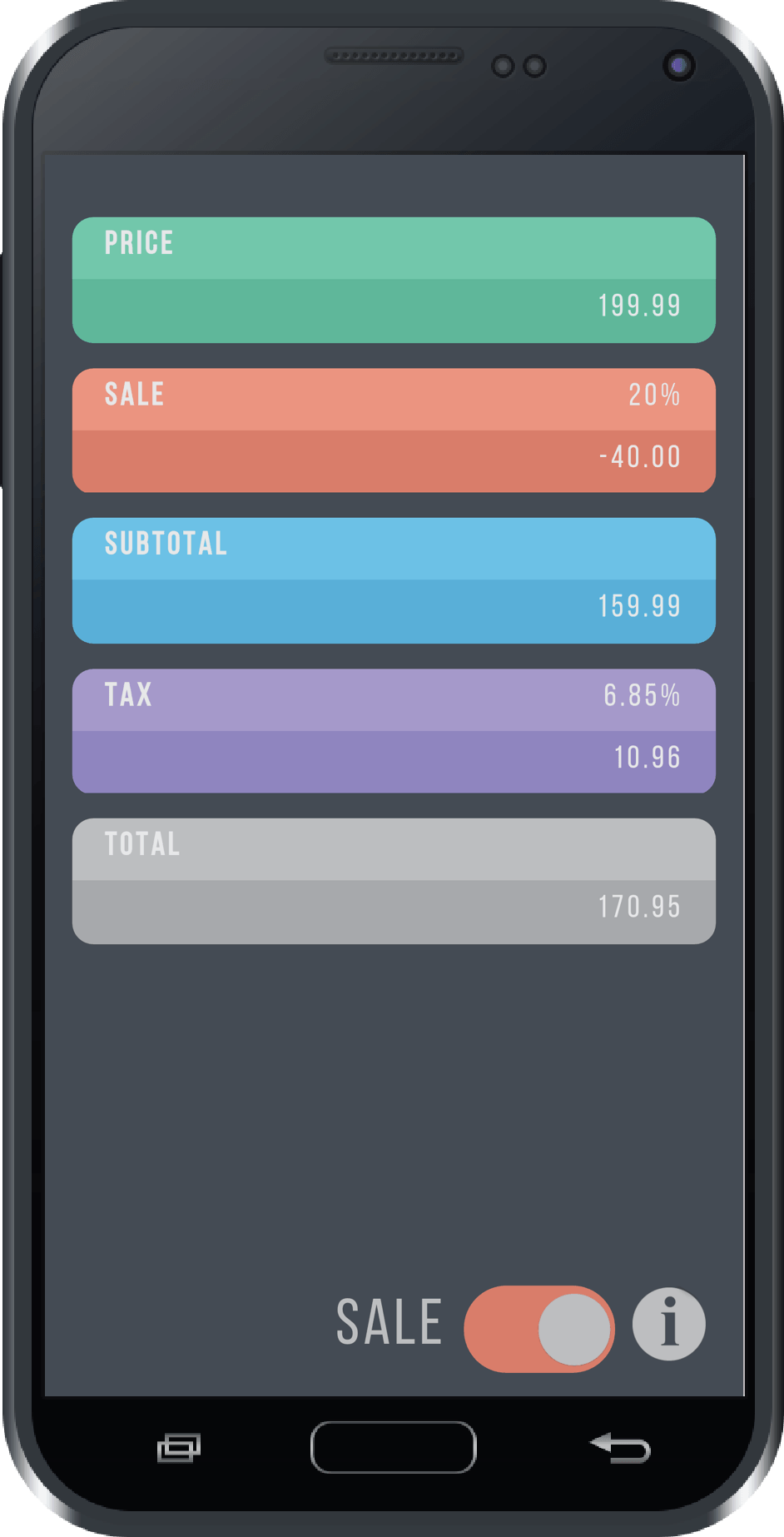# Sales.Tax Calculator

This is a simple and powerful Sales Tax Calculator. Calculate in real time as you enter values. This handy calculator displays price, sales percentage/rate, tax percentage/rate, subtotal, and total price.

FEATURES:

• Calculate values as you enter in real time.
• Calculate sale/discount.
• Calculate from the final total back to the original price.
• Formatted for financial calculations.